Related Articles
How to add a frame to a seaborn heatmap figure in Python?
• Difficulty Level : Easy
• Last Updated : 03 Jan, 2021

A heatmap is a graphical representation of data where values are depicted by color. They make it easy to understand complex data at a glance. Heatmaps can be easily drawn using seaborn in python. In this article, we are going to add a frame to a seaborn heatmap figure in Python.

Syntax: seaborn.heatmap(data, *, vmin=None, vmax=None, cmap=None, center=None, annot_kws=None, linewidths=0, linecolor=’white’, cbar=True, **kwargs)

Important Parameters:

• data: 2D dataset that can be coerced into an ndarray.
• linewidths: Width of the lines that will divide each cell.
• linecolor: Color of the lines that will divide each cell.
• cbar: Whether to draw a colorbar.

All the parameters except data are optional.

Returns: An object of type matplotlib.axes._subplots.AxesSubplot

### Create a heatmap

To draw the heatmap we will use the in-built data set of seaborn. Seaborn has many in-built data sets like titanic.csv, penguins.csv, flights.csv, exercise.csv. We can also make our data set it should just be a rectangular ndarray.

## Python3

 `# Import libraries``import` `seaborn as sns``import` `matplotlib.pyplot as plt`` ` `# Preparing dataset``example ``=` `sns.load_dataset(``"flights"``)``example ``=` `example.pivot(``"month"``, ``"year"``,``                        ``"passengers"``)`` ` `# Creating plot``res ``=` `sns.heatmap(example)`` ` `# show plot``plt.show()`

Output: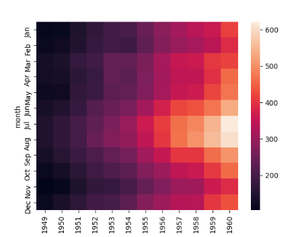basic heatmap

### There are two ways of drawing the frame around a heatmap:

1. Using axhline and axvline.
2. Using spines (more optimal)

Method 1: Using axhline and axvline

The Axes.axhline() and Axes.axvline() function in axes module of matplotlib library is used to add a horizontal and vertical line across the axis respectively.

We can draw two horizontal lines from y=0 and from y= number of rows in our dataset and it will draw a frame covering two sides of our heatmap. Then we can draw two vertical lines from x=0 and x=number of columns in our dataset and it will draw a frame covering the remaining two sides so our heatmap will have a complete frame.

Note: It is not an optimal way to draw a frame as when we increase the line width is does not consider when it is overlapping the heatmap.

Example 1.

## Python3

 `# Import libraries``import` `seaborn as sns``import` `matplotlib.pyplot as plt`` ` `# Preparing dataset``example ``=` `sns.load_dataset(``"flights"``)``example ``=` `example.pivot(``"month"``, ``"year"``,``                        ``"passengers"``)`` ` `# Creating plot``res ``=` `sns.heatmap(example, cmap ``=` `"BuPu"``)`` ` `# Drawing the frame``res.axhline(y ``=` `0``, color``=``'k'``,linewidth ``=` `10``)``res.axhline(y ``=` `example.shape[``1``], color ``=` `'k'``,``            ``linewidth ``=` `10``)`` ` `res.axvline(x ``=` `0``, color ``=` `'k'``,``            ``linewidth ``=` `10``)`` ` `res.axvline(x ``=` `example.shape[``0``], ``            ``color ``=` `'k'``, linewidth ``=` `10``)`` ` `# show plot``plt.show()`

Output: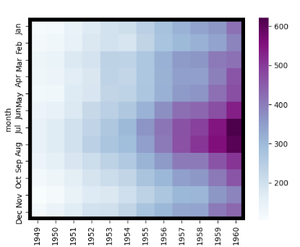Example 2:

## Python3

 `# Import libraries``import` `seaborn as sns``import` `matplotlib.pyplot as plt``import` `numpy as np`` ` `# Preparing dataset``example ``=` `np.random.rand(``10``, ``12``)`` ` `# Creating plot``res ``=` `sns.heatmap(example, cmap ``=` `"magma"``, ``                  ``linewidths ``=` `0.5``)`` ` `# Drawing the frame``res.axhline(y ``=` `0``, color ``=` `'k'``, ``            ``linewidth ``=` `15``)`` ` `res.axhline(y ``=` `10``, color ``=` `'k'``,``            ``linewidth ``=` `15``)`` ` `res.axvline(x ``=` `0``, color ``=` `'k'``,``            ``linewidth ``=` `15``)`` ` `res.axvline(x ``=` `12``, color ``=` `'k'``,``            ``linewidth ``=` `15``)``# show plot``plt.show()`

Output: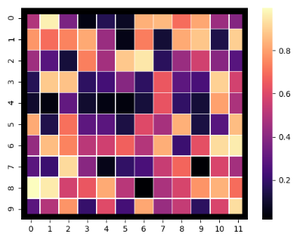Method 2: Using spines

Spines are the lines connecting the axis tick marks and noting the boundaries of the data area. They can be placed at arbitrary positions.

Example 1:

width of the line can be changed using the set_linewidth parameter which accepts a float value as an argument.

## Python3

 `# Import libraries``import` `seaborn as sns``import` `matplotlib.pyplot as plt`` ` `# Preparing dataset``example ``=` `sns.load_dataset(``"flights"``)``example ``=` `example.pivot(``"month"``, ``"year"``, ``                        ``"passengers"``)`` ` `# Creating plot``res ``=` `sns.heatmap(example, cmap ``=` `"Purples"``)`` ` `# Drawing the frame``for` `_, spine ``in` `res.spines.items():``    ``spine.set_visible(``True``)``    ``spine.set_linewidth(``5``)`` ` `# show plot``plt.show()`

Output: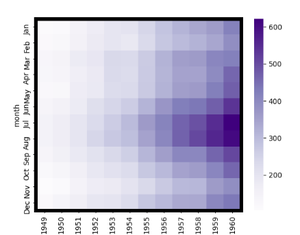Example 2:

We can specify the style of the frame using the set_linestyle parameter of the spine(solid, dashed, dashdot, dotted).

## Python3

 `# Import libraries``import` `seaborn as sns``import` `matplotlib.pyplot as plt``import` `numpy as np`` ` `# Preparing dataset``example ``=` `np.random.rand(``10``, ``12``)`` ` `# Creating plot``res ``=` `sns.heatmap(example, cmap ``=` `"Greens"``,``                  ``linewidths ``=` `2``,``                  ``linecolor ``=` `"white"``)`` ` `# Drawing the frame``for` `_, spine ``in` `res.spines.items():``    ``spine.set_visible(``True``)``    ``spine.set_linewidth(``3``)``    ``spine.set_linestyle(``"dashdot"``)`` ` `# show plot``plt.show()`

Output: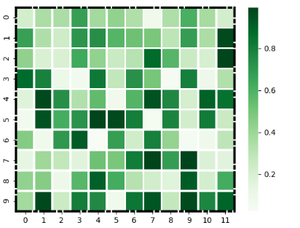Attention geek! Strengthen your foundations with the Python Programming Foundation Course and learn the basics.

To begin with, your interview preparations Enhance your Data Structures concepts with the Python DS Course.

My Personal Notes arrow_drop_up